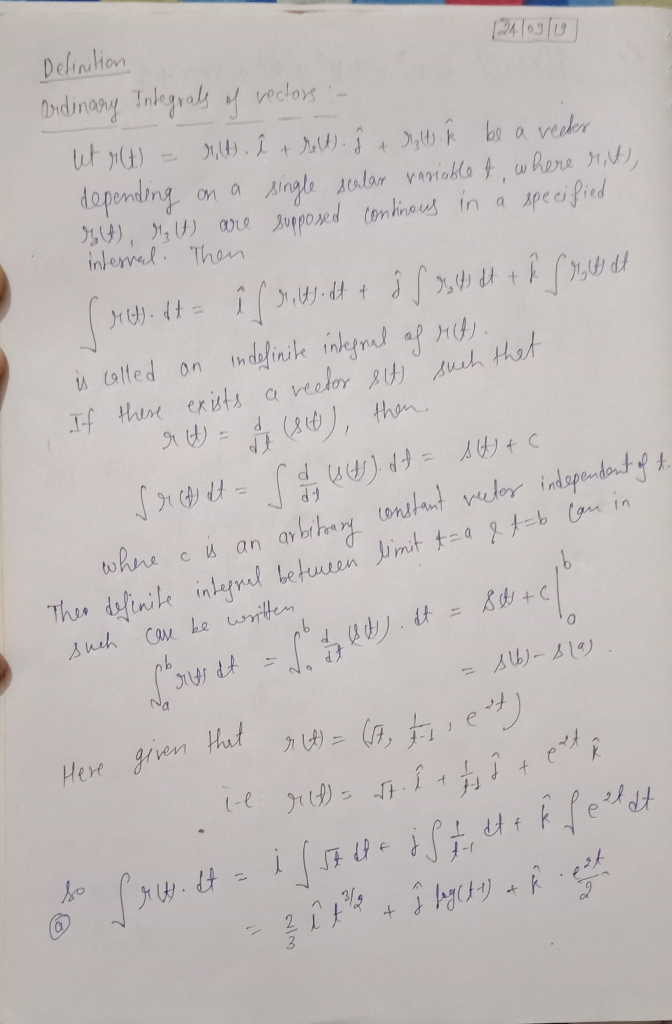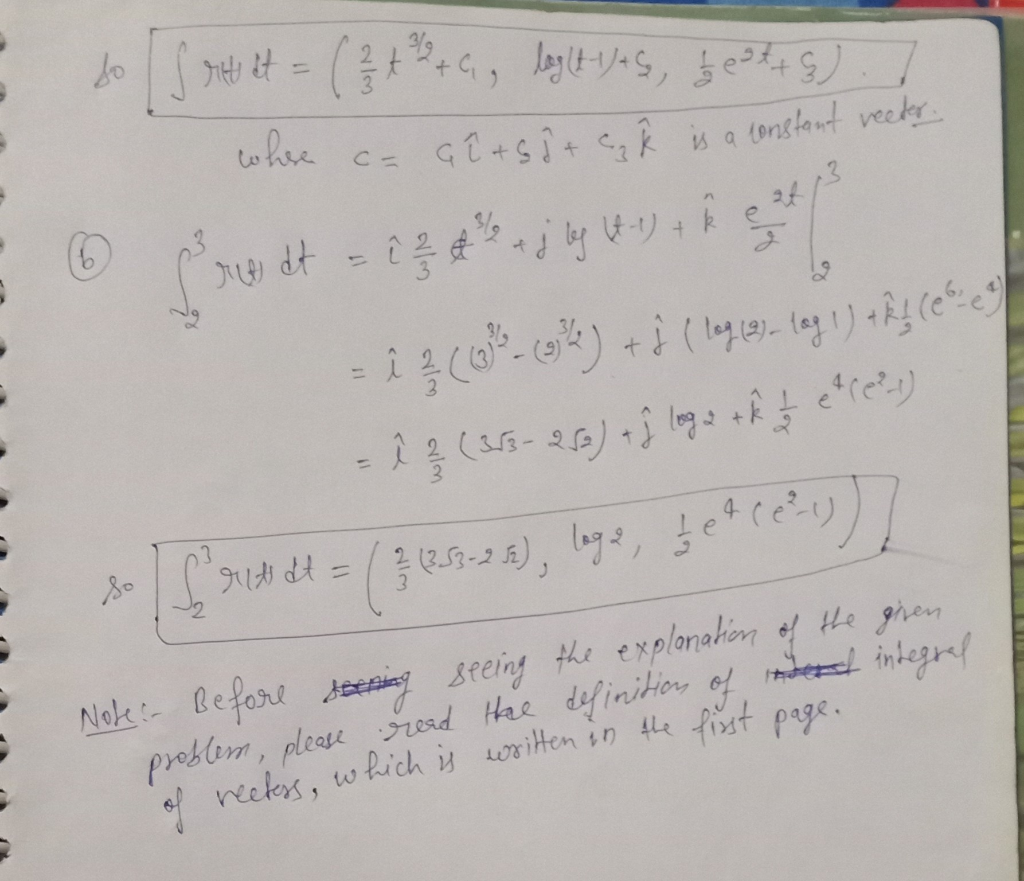# Given the vector function r(t)=〈√t , 1/(t-1) ,e^2t 〉 a) Find: ∫ r(t)dt b) Calculate the...

Given the vector function r(t)=〈√t , 1/(t-1) ,e^2t 〉 a) Find: ∫ r(t)dt b) Calculate the definite integral of r(t) for 2 ≤ t ≤ 3

can you please provide a Matlab code?

## Solutions

##### Expert Solution## Related Solutions

##### Consider the vector function given below. r(t) = 2t, 3 cos(t), 3 sin(t) (a) Find the...
Consider the vector function given below. r(t) = 2t, 3 cos(t), 3 sin(t) (a) Find the unit tangent and unit normal vectors T(t) and N(t). T(t)   =    N(t)   =    (b) Use this formula to find the curvature. κ(t) =
##### what is the value of ∫∞−∞ δ(t+2)e^(−2t)u(t)dt?
what is the value of ∫∞−∞ δ(t+2)e^(−2t)u(t)dt?
##### Consider the helix r(t)=(cos(2t),sin(2t),−3t)r(t)=(cos(2t),sin(2t),−3t). Compute, at t=π/6 A. The unit tangent vector T=T= ( , ,...
Consider the helix r(t)=(cos(2t),sin(2t),−3t)r(t)=(cos(2t),sin(2t),−3t). Compute, at t=π/6 A. The unit tangent vector T=T= ( , , ) B. The unit normal vector N=N= ( , , ) C. The unit binormal vector B=B= ( , , ) D. The curvature κ=κ=
##### How to find the unit vectors for the following equation: r(t) = <e^t,2e^-t,2t> A) Compute the...
How to find the unit vectors for the following equation: r(t) = <e^t,2e^-t,2t> A) Compute the unit Tangent Vector, unit Normal Vector, and unit Binomial Vector. B) Find a formula for k, the curvature. C) Find the normal and osculating planes at t=0
##### Let r(t) = 2t ,4t2 ,2t be a position function for some object. (a) (2 pts)...
Let r(t) = 2t ,4t2 ,2t be a position function for some object. (a) (2 pts) Find the position of the object at t = 1. (b) (6 pts) Find the velocity of the object at t = 1. (c) (6 pts) Find the acceleration of the object at t = 1. (d) (6 pts) Find the speed of the object at t = 1. (e) (15 pts) Find the curvature K of the graph C determined by r(t) when...
##### Find the curvature of the parametrized curve ~r(t) =< 2t 2 , 4 + t, −t...
Find the curvature of the parametrized curve ~r(t) =< 2t 2 , 4 + t, −t 2 >.
##### Find the general solution: dx/dt + x/(1+2t) = 5
Find the general solution: dx/dt + x/(1+2t) = 5
##### FOR THE PARAMETRIZED PATH r(t)= e^tcos(πt)i+e^tsin(πt)j+e^tk a) find the velocity vector, the unit tangent vector and...
FOR THE PARAMETRIZED PATH r(t)= e^tcos(πt)i+e^tsin(πt)j+e^tk a) find the velocity vector, the unit tangent vector and the arc lenght between t=0 and t=1 b) find a point where the path given by r(t) intersects the plane x-y=0 and determine the angle of intersection between the tangent vector to the curve and the normal vector to the plane.
##### Given r(t) = <2 cos(t), 2 sin(t), 2t>. • What is the arc length of r(t)...
Given r(t) = <2 cos(t), 2 sin(t), 2t>. • What is the arc length of r(t) from t = 0 to t = 5. SET UP integral but DO NOT evaluate • What is the curvature κ(t)?
##### (1 point) For the given position vectors r(t)r(t) compute the unit tangent vector T(t)T(t) for the...
(1 point) For the given position vectors r(t)r(t) compute the unit tangent vector T(t)T(t) for the given value of tt . A) Let r(t)=〈cos5t,sin5t〉 Then T(π4)〈 B) Let r(t)=〈t^2,t^3〉 Then T(4)=〈 C) Let r(t)=e^(5t)i+e^(−4t)j+tk Then T(−5)=